# NCERT Solutions For Class 10 Maths Chapter 13 Exercise 13.4

## Chapter 13 Ex.13.4 Question 1

A drinking glass is in the shape of a frustum of a cone of height $$14\,\rm{cm.}$$ The diameters of its two circular ends are $$4\,\rm{cm}$$ and $$2\,\rm{cm.}$$ Find the capacity of the glass.

### Solution

What is Known?

A drinking glass is in the shape of a frustum of a cone of height is  $$14\, \rm{cm}.$$

The diameter of its two circular ends are $$4\, \rm{cm}$$ and $$2\,\rm{cm}.$$

What is Unknown?

The capacity of the glass

Reasoning:

Draw a figure to visualize the shape better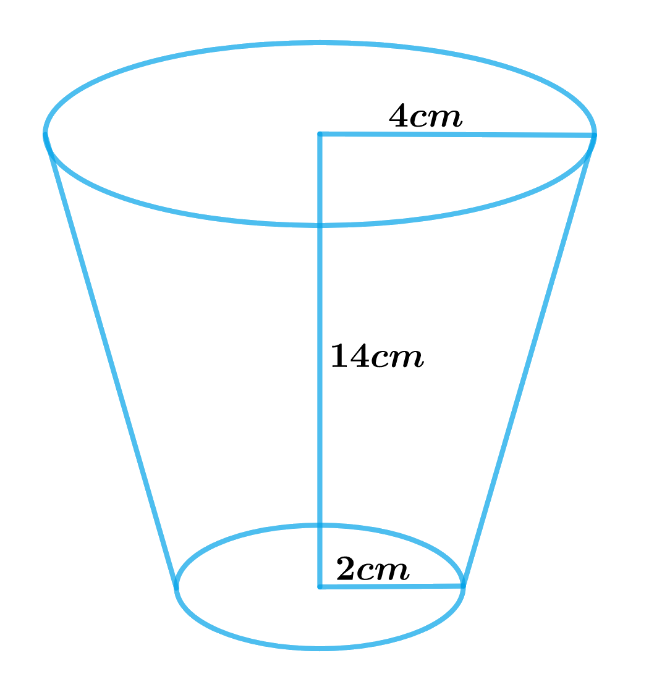Since the glass is in the shape of a frustum of a cone

Therefore, the capacity of the glass $$=$$ Volume of frustum of a cone

We will find the capacity of the glass by using formulae

Volume of frustum of a cone\begin{align} = \frac{1}{3}\pi h\left( {r_1^2 + r_2^2 + {r_1}{r_2}} \right)\end{align}

where $$r1, r2$$ and $$h$$ are the radii and height of the frustum of the cone respectively.

Steps:

Height of glass,$$h = 14 \rm cm$$

Radius of the larger base,\begin{align} {r_1} = \frac{{4 \rm cm}}{2} = 2 \rm cm\end{align}

Radius of the smaller base,\begin{align} {r_2} = \frac{{2 \rm cm}}{2} = 1 \rm cm\end{align}

The capacity of the glass $$=$$ Volume of frustum of a cone

\begin{align}&= \frac{1}{3}\pi h\left( {r_1^2 + r_2^2 + {r_1}{r_2}} \right)\\&= \begin{bmatrix} \frac{1}{3} \times \frac{{22}}{7} \times 14 \rm cm \times \\ \begin{pmatrix} \left( 2 \rm cm \right)^2 + \left( 1 \rm cm \right)^2 \\ + 2 \rm cm \times 1cm \end{pmatrix} \end{bmatrix} \\&= \begin{bmatrix} \frac{{44}}{3} \rm cm \times\\ \begin{pmatrix} 4 \rm c{m^2} + 1c{m^2} \\ + 2 \rm c{m^2} \end{pmatrix} \end{bmatrix} \\&= \frac{{44}}{3} \rm cm \times 7 \rm c{m^2}\\&= \frac{{308}}{3} \rm c{m^2}\\&= 102\frac{2}{3} \rm c{m^2}\end{align}

Therefore, the capacity of the glass is \begin{align}102\frac{2}{3}\,\rm{cm^3}\end{align}

## Chapter 13 Ex.13.4 Question 2

The slant height of a frustum of a cone is $$4\,\rm{cm}$$ and the perimeters (circumference) of its circular ends are $$18 \,\rm{cm}$$ and $$6\, \rm{cm.}$$ Find the curved surface area of the frustum.

### Solution

What is Known?

Slant height of a frustum of a cone is $$4\,\rm{cm}$$ and the circumference of its circular ends are $$18\, \rm{cm}$$ and $$6 \,\rm{cm.}$$

What is unknown?

The curved surface area of the frustum.

Reasoning:

Draw a figure to visualize the shape better

Using the circumferences of the circular ends of the frustum to find the radii of its circular ends

Circumference of the circle $$= 2\pi r$$

where $$r$$ is the radius of the circle.

We will find the CSA of the frustum by using formula;

CSA of frustum of a cone $$= \pi \left( {{r_1} + {r_2}} \right)l$$

where $$r1, r2$$ and $$l$$ are the radii and slant height of the frustum of the cone respectively.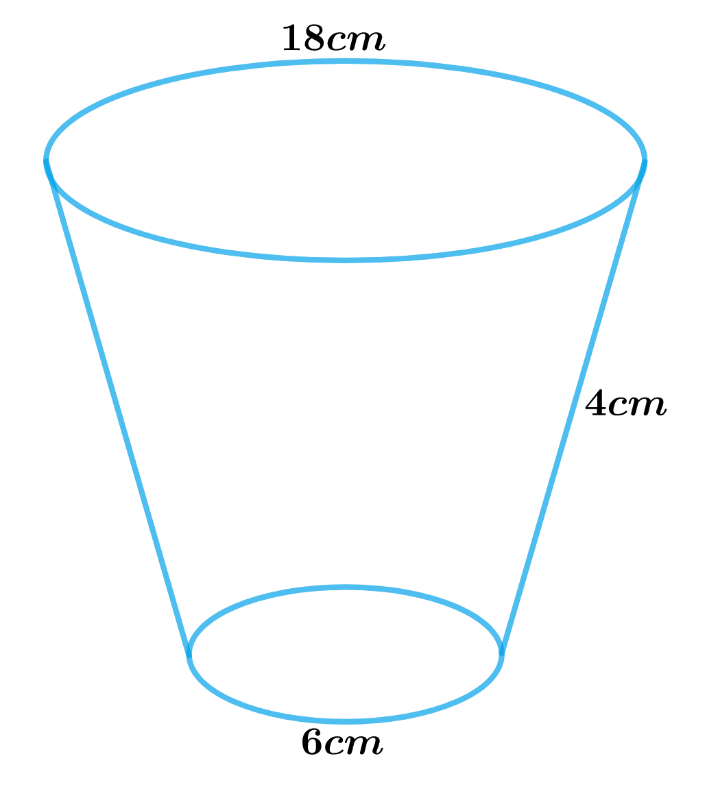Steps:

Slant height of frustum of a cone, $$l = 4 \rm cm$$

Circumference of the larger circular end, $${C_1} = 18 \rm cm$$

Radius of the larger circular end,\begin{align} {r_1} = \frac{{{C_1}}}{{2\pi }} = \frac{{18 \rm cm}}{{2\pi }} = \frac{9}{\pi } \rm cm\end{align}

Circumference of the smaller circular end, $${C_2} = 6 \rm cm$$

Radius of the smaller circular end,\begin{align} {r_2} = \frac{{{C_2}}}{{2\pi }} = \frac{{6 \rm cm}}{{2\pi }} = \frac{3}{\pi } \rm cm\end{align}

CSA of frustum of a cone $$= \pi \left( {{r_1} + {r_2}} \right)l$$

\begin{align}&= \pi \left( {\frac{9}{\pi } \rm cm + \frac{3}{\pi } \rm cm} \right) \times 4 \rm cm\\&= \pi \times \frac{{12}}{\pi } \rm cm \times 4cm\\&= 48 \rm c{m^2}\end{align}

The curved surface area of the frustum is $$48 \;\rm cm^2.$$

## Chapter 13 Ex.13.4 Question 3

A fez, the cap used by the Turks, is shaped like the frustum of a cone (see Fig. 13.24). If its radius on the open side is $$10\,\rm{cm}$$, radius at the upper base is $$4\,\rm{cm}$$ and its slant height is $$15\,\rm{cm, }$$ find the area of material used for making it.

### Solution

What is Known?

A fez is shaped like the frustum of a cone with radius of open side $$10\,\rm{cm},$$

radius at the upper base $$4\,\rm{cm}$$ and Slant Height $$15 \,\rm{cm}$$

What is Unknown?

Area of the material used for making Fez

Reasoning: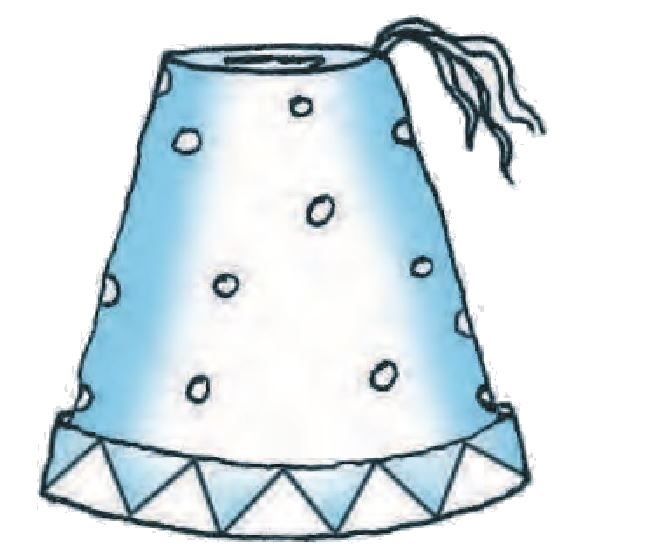Since the fez is in the shape of frustum of a cone and is open at the bottom.

Therefore,

Area of material used for making fez $$=$$ Curved Surface Area of the frustum $$+$$ Area of the upper circular end

We will find the Area of material by using formulae;

CSA of frustum of a cone $$= \pi \left( {{r_1} + {r_2}} \right)l$$

where $$r1, r2$$ and $$l$$ are the radii and slant height of the frustum of the cone respectively.

Area of the circle $$= \pi {r^2}$$

where $$r$$ is the radius of the circle.

Steps:

Slant height, $$l =15\,\rm{cm}$$

Radius of open side $$r_1=10\,\rm{cm}$$

Radius of upper base $${r_2} =4\,\rm{cm}$$

Area of material used for making fez $$=$$ Curved Surface area of the frustum $$+$$ area of the upper circular end

\begin{align}&= \pi \left( {{r_1} + {r_2}} \right)l + \pi {r^2}\\&= \pi \left[ {\left( {{r_1} + {r_2}} \right)l + r_2^2} \right]\end{align}

\begin{align}&=\!\frac{{22}}{7}\left[\!{\left( {10cm\!+\!4cm} \right)\!15cm\!+\!\!{{\left( {4cm} \right)}\!^2}}\!\right]\\&= \frac{{22}}{7}\left[ {14cm \times 15cm + 16c{m^2}} \right]\\&= \frac{{22}}{7}\left[ {210c{m^2} + 16c{m^2}} \right]\\&= \frac{{22}}{7} \times 226c{m^2}\\&= \frac{{4972}}{7}c{m^2}\\&= 710\frac{2}{7}c{m^2}\end{align}

\begin{align} 710\frac{2}{7}c{m^2}\end{align} of the material used for making Fez.

## Chapter 13 Ex.13.4 Question 4

A container, opened from the top and made up of a metal sheet, is in the form of a frustum of a cone of height $$16 \,\rm{cm}$$ with radii of its lower and upper ends as $$8 \,\rm{cm}$$ and $$20 \,\rm{cm}$$, respectively. Find the cost of the milk which can completely fill the container, at the rate of  $$₹\,20$$ per liter. Also find the cost of metal sheet used to make the container, if it costs  $$₹\,8$$ per $$100 \rm \,cm^2.$$

$$(\rm{Take}\, \pi = 3.14)$$

### Solution

What is Known?

A container opened from the top and made up of a metal sheet is in the shape of a frustum of a cone with of height $$16\rm{ cm}$$, radius of lower end $$8\rm{ cm}$$ and radius of upper end $$20 \rm{cm.}$$

Cost of $$1$$ litre of milk is $$₹ 20$$ and Cost of $$100\,\rm{cm^2}$$ of metal sheet is $$₹ 8.$$

What is Unknown?

Cost of the milk which can completely fill the container and Cost of metal sheet used to make the container

Reasoning: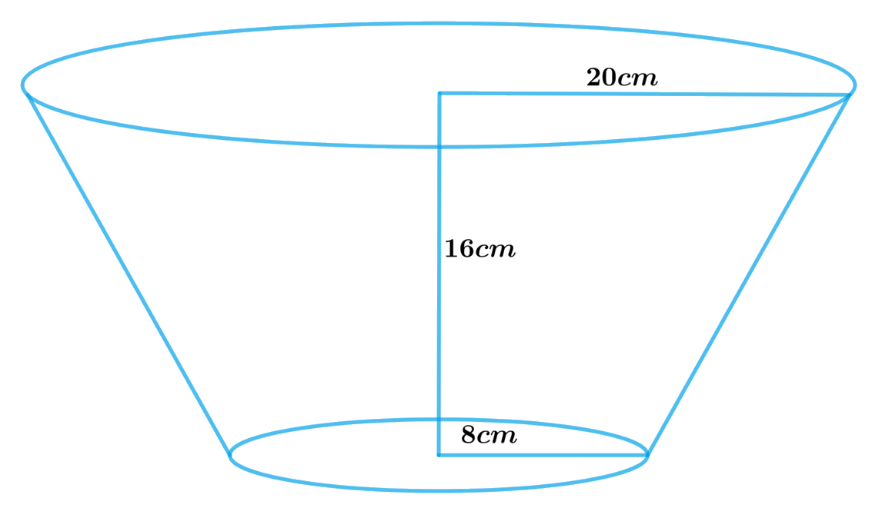To find the cost of milk:

Since the container is in the shape of a frustum of a cone, so volume of milk which can completely fill the container will be equal to volume of frustum of cone.

Therefore, volume of milk $$=$$ volume of frustum of cone

We will find the volume of the frustum by using formula;

Volume of frustum of a cone\begin{align} = \frac{1}{3}\pi h\left( {r_1^2 + r_2^2 + {r_1}{r_2}} \right)\end{align}

where $$r1, r2$$ and $$h$$ are the radii and height of the frustum of the cone respectively.

Once we know the volume of milk, we need to convert it into litre as the cost of milk is in terms of $$₹$$ per litre.

\begin{align}1{\text{ }}litre &= 1000c{m^3}\\1c{m^3} &= \frac{1}{{1000}}\text{litre}\end{align}

To find the cost of metal sheet:

Since the container is open from the top the metal sheet will only contain CSA of frustum of the cone and area of the lower circular end.

Therefore,

Area of metal sheet required to make the container $$=$$ CSA of frustum of the cone $$+$$ Area of lower circular end

We will find the CSA of the frustum by using formulae;

CSA of frustum of a cone$$= \pi \left( {{r_1} + {r_2}} \right)l$$

Slant height\begin{align}l = \sqrt {{{\left( {{r_1} - {r_2}} \right)}^2} + {h^2}} \end{align} $$(r1 > r2)$$

where $$r1, r2,h$$ and $$l$$ are the radii height and slant height of the frustum of the cone respectively.

Area of the lower circular end $$= \pi r_2^2$$

where $$r2$$ is the radius of the lower circular end.

Steps:

Height of the frustum of cone, $$h =16 \,\rm{cm}$$

Radius of the upper end, $${r_1}=20 \,\rm{cm}$$

Radius of the lower end, $${r_2}=8\;\rm{cm}$$

Slant height of the frustum,

\begin{align}l &= \sqrt {{{\left( {{r_1} - {r_2}} \right)}^2} + {h^2}}\\l &= \sqrt {{{\left( {20cm - 8cm} \right)}^2} + {{\left( {16cm} \right)}^2}} \\&= \sqrt {144c{m^2} + 256c{m^2}} \\&= \sqrt {400c{m^2}} \\&= 20cm\end{align}

Volume of milk which can completely fill the container $$=$$ Volume of the frustum of Cone

\begin{align}&= \frac{1}{3}\pi h\left( {r_1^2 + r_2^2 + {r_1}{r_2}} \right)\\&= \frac{1}{3} \times 3.14 \times 16cm \times \left( {{{\left( {20cm} \right)}^2} + {{\left( {8cm} \right)}^2} + 20cm \times 8cm} \right)\\&= \frac{1}{3} \times 3.14 \times 16cm \times \left( {400c{m^2} + 64c{m^2} + 160c{m^2}} \right)\\&= \frac{1}{3} \times 3.14 \times 16cm \times 624c{m^2}\\&= 10449.92c{m^3}\\&= \frac{{10449.92}}{{1000}}\,\text{litre}\\&= 10.44992\,\text{litre}\\&= 10.45\,\text{litre}\end{align}

Cost of $$1$$ lite of milk \begin{align}=₹ \;20\end{align}

Therefore, cost of $$10.45$$ liter of milk \begin{align} = 20 \times 10.45 = \,₹\, 209 \end{align}

Area of metal sheet required to make the container $$=$$ CSA of frustum of the cone $$+$$ Area of lower circular end

\begin{align} & =\pi \left( {{r}_{1}}+{{r}_{2}} \right)l+\pi r_{2}^{2} \\ & =\pi \left[ \left( {{r}_{1}}+{{r}_{2}} \right)l+r_{2}^{2} \right] \\ & =3.14\times \left[ \begin{array} & \left( 20cm+8cm \right)\times \\ 20cm+{{\left( 8cm \right)}^{2}} \\ \end{array} \right] \\ & =3.14\times \left[ 560c{{m}^{2}}+64c{{m}^{2}} \right] \\ & =3.14\times 624c{{m}^{2}} \\ & =1959.36c{{m}^{2}} \\ \end{align}

Cost of $$100 \rm cm^2$$ of metal sheet $$= \,₹\;8$$

Therefore, cost of $$1959.36c{m^2}$$ of metal sheet \begin{align}= ₹ \frac{8}{{100}} \times 1959.36\end{align}

\begin{align}&= ₹ 156.7488\\ &= ₹ 156.75\end{align}

Cost of milk which can completely fill the container is $$₹\;209$$

Cost of metal sheet required to make the container is $$₹\; 156.75$$

## Chapter 13 Ex.13.4 Question 5

A metallic right circular cone $$20\,\rm{cm}$$ high and whose vertical angle is $$60^\circ$$ is cut into two parts at the middle of its height by a plane parallel to its base. If the frustum so obtained be drawn into a wire of diameter \begin{align}\frac{1}{{16}}\;\rm{cm}\end{align} Find the length of the wire.

### Solution

What is Known?

(i) A metallic right circular cone of height $$=20\;\rm{cm}$$ and whose vertical angle $$= 60^\circ$$ is cut into $$2$$ parts at the middle of its height by a plane parallel to its base

(ii) The frustum obtained is drawn into a wire of diameter \begin{align}=\frac{1}{{16}}\;\rm{cm}\end{align}

What is Unknown?

Length of the wire drawn out from a frustum

Reasoning:

Draw a figure to visualize the vertical cross section of a cone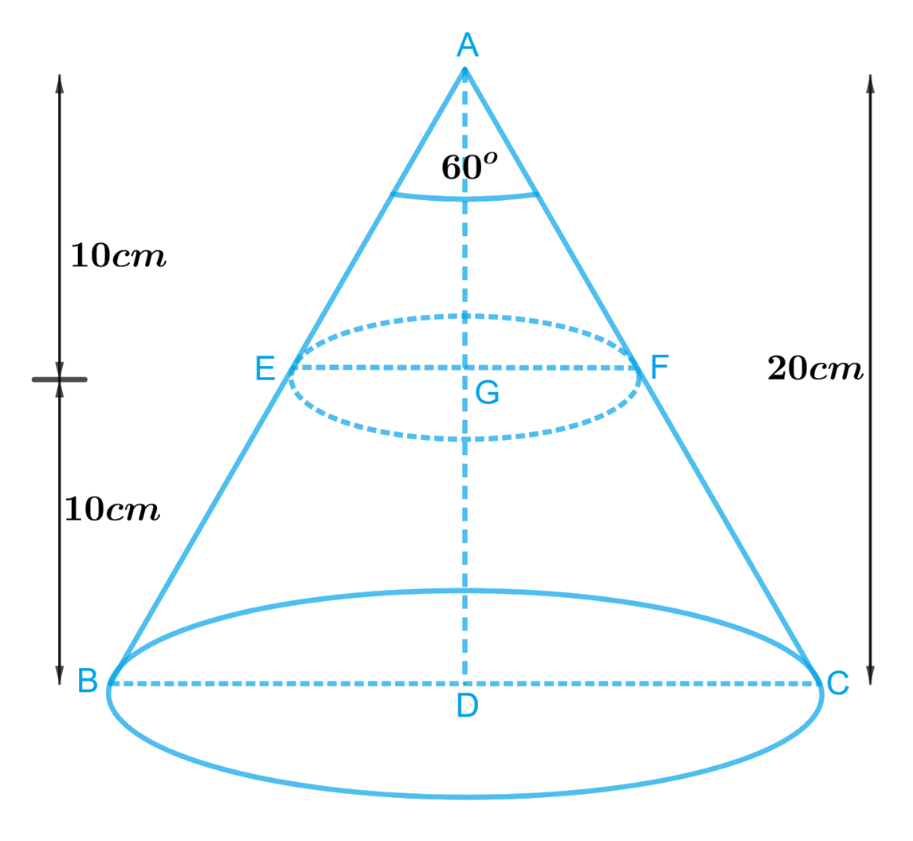Since a metallic right circular cone is cut into two parts at the middle of its height by a plane parallel to its base.

From the figure it’s clear that

In , $$\Delta ABC$$ , $$EF$$ parallel to $$BC$$ and

\begin{align}AD &= 20cm\\AG &= 10cm\\\angle BAC &= 60^\circ \end{align}

To get the values of radii of both ends of the frustum formed compare $$\Delta ADB$$ and $$\Delta ADC$$

\begin{align}AD &= AD\,\left ( {{\text{Common}}} \right)\\AB &= AC\,\left( {{\text{Slant height}}} \right)\\\angle ADB &= \angle ADC = {90^{\rm{o}}}{\rm{ }}\,\, \left( {{\text{Right circular cone}}} \right)\\\Delta ADB &\cong \Delta ADC\, \left( {{\text{RHS criterion of congruency}}} \right)\\\angle BAD &= \angle DAC\,\left( {{\text{CPCT}}}\right)\end{align}

Then,

\begin{align}\angle BAD &= \angle DAC \\&= \frac{1}{2}\angle BAC \\&= \frac{1}{2} \times 60^\circ = 30^\circ \end{align}

In $$\Delta ADB$$

\begin{align}\frac{{BD}}{{AD}} &= \tan 30^\circ \\BD &= AD\tan 30^\circ \end{align}

Radius of lower end \begin{align}BD = \frac{{AD}}{{\sqrt 3 }}\end{align}

Similarly, in $$\Delta AEG$$

\begin{align}\frac{{EG}}{{AG}} &= \tan 30^\circ \\EG &= AG\tan 30^\circ \end{align}

Radius of upper end \begin{align}EG = \frac{{AG}}{{\sqrt 3 }}\end{align}

Now radii and height are known, finding volume of frustum of cone is easy

Since the frustum obtained is drawn into the wire which will be cylindrical in the shape.

Then the volume of the wire will be same as the volume of the frustum of the cone.

Therefore, Volume of the wire $$=$$ Volume of frustum of the cone

We will find the volume of the frustum by using formula;

Volume of frustum of a cone \begin{align} = \frac{1}{3}\pi h\left( {r_1^2 + r_2^2 + {r_1}{r_2}} \right)\end{align}

where $$r1, r2$$ and $$h$$ are the radii and height of the frustum of the cone respectively.

We will find the volume of the wire by using formula;

Volume of cylinder $$= \pi {r^2}h$$

where $$r$$ and $$h$$ are radius and height of the cylinder.

Steps:

In ,$$\Delta ABC$$ $$EF$$ parallel to $$BC$$ and

\begin{align}AD &= 20cm\\AG &= 10cm\\\angle BAC &= 60^\circ \end{align}

To get the values of radii of both ends of the frustum formed compare $$\Delta ADB$$ and $$\Delta ADC$$

\begin{align}AD &= AD\,\left ( {{\text{Common}}} \right)\\AB &= AC\,\left( {{\text{Slant height}}} \right)\\\angle ADB &= \angle ADC = {90^{\rm{o}}}{\rm{ }}\,\, \left( {{\text{Right circular cone}}} \right)\\\Delta ADB &\cong \Delta ADC\, \left( {{\text{RHS criterion of congruency}}} \right)\\\angle BAD &= \angle DAC\,\,\,\left( {{\text{CPCT}}}\right)\end{align}

Then,

\begin{align}\angle BAD &= \angle DAC \\&= \frac{1}{2}\angle BAC\\& = \frac{1}{2} \times 60^\circ = 30^\circ \end{align}

In $$\Delta ADB$$

\begin{align}\frac{{BD}}{{AD}} &= \tan 30^\circ \\BD &= AD\tan 30^\circ \\BD &= 20cm \times \frac{1}{{\sqrt 3 }}\\BD &= \frac{{20\sqrt 3 }}{3}cm\end{align}

Similarly, in $$\Delta AEG$$

\begin{align}\frac{{EG}}{{AG}} &= \tan 30^\circ \\EG &= AG\tan 30^\circ \\EG &= 10cm \times \frac{1}{{\sqrt 3 }}\\EG &= \frac{{10\sqrt 3 }}{3}cm\end{align}

Height of the frustum of the cone,$$h = 10cm$$

Radius of lower end,\begin{align}{r_1} = \frac{{20\sqrt 3 }}{3}cm\end{align}

Radius of upper end,\begin{align}{r_2} = \frac{{10\sqrt 3 }}{3}cm\end{align}

Diameter of the cylindrical wire,\begin{align}d = \frac{1}{{16}}cm\end{align}

Radius of the cylindrical wire,\begin{align}r = \frac{1}{2} \times \frac{1}{{16}}cm = \frac{1}{{32}}cm\end{align}

Let the length of the wire be $$H$$

Since the frustum is drawn into wire

Volume of the cylindrical wire $$=$$ Volume of frustum of the cone

\begin{align}\pi {r^2}H &= \frac{1}{3}\pi h\left( {r_1^2 + r_2^2 + {r_1}{r_2}} \right)\\H &= \frac{h}{{3{r^2}}}\left( {r_1^2 + r_2^2 + {r_1}{r_2}} \right)\\&= \frac{{10cm}}{{3 \times {{\left( {\frac{1}{{32}}cm} \right)}^2}}} \times \left[ {{{\left( {\frac{{20\sqrt 3 }}{3}cm} \right)}^2} + {{\left( {\frac{{10\sqrt 3 }}{3}cm} \right)}^2} + \frac{{20\sqrt 3 }}{3}cm \times \frac{{10\sqrt 3 }}{3}cm} \right]\\&= \frac{{10240}}{{3cm}} \times \left[ {\frac{{400}}{3}c{m^2} + \frac{{100}}{3}c{m^2} + \frac{{200}}{3}c{m^2}} \right]\\&= \frac{{10240}}{{3cm}} \times \frac{{700c{m^2}}}{3}\\&= \frac{{7168000}}{{9 \times 100}}m\\&= 7964.4m\end{align}

Length of the wire is $$7964.4m$$

Download SOLVED Practice Questions of NCERT Solutions For Class 10 Maths Chapter 13 Exercise 13.4 for FREE
Ncert Class 10 Exercise 13.4
Ncert Solutions For Class 10 Maths Chapter 13 Exercise 13.4
Instant doubt clearing with Cuemath Advanced Math Program